## Classification

#### Classification

Direction: In following questions select the one which is different from the other three responses.

1. NA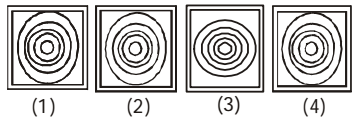1. In figure (3) all circles are concentric.

##### Correct Option: C

In figure (3) all circles are concentric. But all other figure are in same .

Direction: In each of the following questions select the one which is different from the other three responses.

1. NA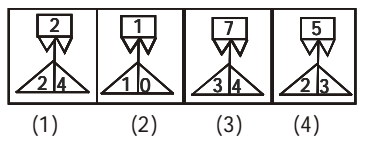1. Except one figure , all others the top number is the sum of the two bottom numbers .

##### Correct Option: A

Except in figure (1) in all others the top number is the sum of the two bottom numbers .

1. NA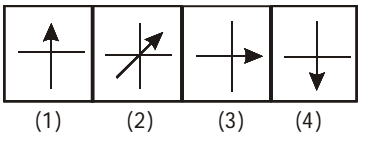1. All other figures one line segment intersects one arrow at right angle.

##### Correct Option: B

Except in figure (2), in all other figures one line segment intersects one arrow at right angle.

1. NA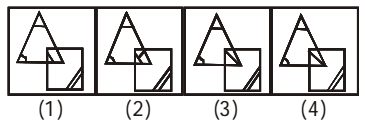1. In figure (2) there is one more line segment than the other figures.

##### Correct Option: B

In figure (2) there is one more line segment than the other figures. But all other figures are in same .

1. NA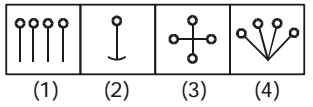1. Except one figure, in all other figures there are four line segments with small circle.

##### Correct Option: B

Except in figure (2), in all other figures there are four line segments with small circle. But all other figures are different to figure ( 2 ) .